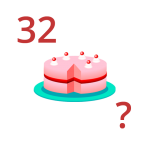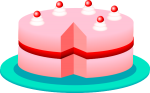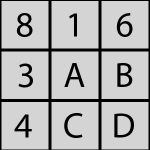Puzzle Command
Daily Puzzles
LogicFind the correct number in this logic puzzle.Find the correct number in this logic puzzle.Find the correct number in this logic puzzle.Find the correct number in this logic puzzle.Find the correct number in this logic puzzle.Find the correct number in this logic puzzle.David and Larry are discussing Latisha's age. Help David solve the math problem.An octagon has eight sides. Can you solve this simple question?Find the correct number in this logic puzzle.Latisha has baked a beautiful cake. When she comes home from her book study she finds it has been eaten.Find the correct number in this logic puzzle.A series of numbers are arranged in a matrix. Solve for A.Find the correct number in this logic puzzle.Find the correct number in this logic puzzle.Find the correct number in this logic puzzle.Find the correct number in this logic puzzle.Find the correct number in this logic puzzle.Find the correct number in this logic puzzle.Find the correct number in this logic puzzle.Find the correct number in this logic puzzle.Find the correct number in this logic puzzle.Find the correct number in this logic puzzle.Find the correct number in this logic puzzle.Find the correct number in this logic puzzle.Find the correct number in this logic puzzle.Find the correct number in this logic puzzle.Charlie and Peter have found some contraband while working Airport Security. Help them figure out who the culprit is.Find the correct number in this logic puzzle.Find the correct number in this logic puzzle.Find the correct number in this logic puzzle.Find the correct number in this logic puzzle.